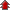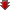Home Board Paper Solutions Text Book Solutions Articles NCERT Exemplar Solutions

# The d-and f-Block Elements### Exercise 1

•  Q1 Silver atom has completely filled d orbitals (4d10) in its ground state. How can you say that it is a transition element? Q2 In the series Sc (Z = 21) to Zn (Z = 30), the enthalpy of atomization of zinc is the lowest, i.e., 126 kJ mol−1. Why? Q3 Which of the 3d series of the transition metals exhibits the largest number of oxidation states and why? Q4 The Eθ(M2+/M) value for copper is positive (+0.34V). What is possibly the reason for this? (Hint: consider its high ΔaHV and low ΔhydHV) Q5 How would you account for the irregular variation of ionization enthalpies (first and second) in the first series of the transition elements? Q6 Why is the highest oxidation state of a metal exhibited in its oxide or fluoride only? Q7 Which is a stronger reducing agent Cr2+ or Fe2+ and why? Q8 Calculate the 'spin only' magnetic moment of M2+(aq) ion (Z = 27). Q9 Explain why Cu+ ion is not stable in aqueous solutions? Q10 Actinoid contraction is greater from element to element than lanthanoid contraction. Why?### Exercise 2

•  Q1 Q1 : Write down the electronic configuration of: (i) Cr3+    (iii) Cu+    (v) Co2+    (vii) Mn2+  (ii) Pm3+  (iv) Ce4+  (vi) Lu2+   (viii) Th4+ Q2 Why are Mn2+compounds more stable than Fe2+ towards oxidation to their +3 state? Q3 Explain briefly how +2 state becomes more and more stable in the first half of the first row transition elements with increasing atomic number?">Explain briefly how +2 state becomes more and more stable in the first half of the first row transition elements with increasing atomic number? Q4 To what extent do the electronic configurations decide the stability of oxidation states in the first series of the transition elements? Illustrate your answer with examples.">To what extent do the electronic configurations decide the stability of oxidation states in the first series of the transition elements? Illustrate your answer with examples. Q5 What may be the stable oxidation state of the transition element with the following d electron configurations in the ground state of their atoms : 3d3, 3d5, 3d8 and 3d4?">What may be the stable oxidation state of the transition element with the following d electron configurations in the ground state of their atoms : 3d3, 3d5, 3d8 and 3d4? Q6 Name the oxometal anions of the first series of the transition metals in which the metal exhibits the oxidation state equal to its group number.">Name the oxometal anions of the first series of the transition metals in which the metal exhibits the oxidation state equal to its group number. Q7 What is lanthanoid contraction? What are the consequences of lanthanoid contraction? Q8 What are the characteristics of the transition elements and why are they called transition elements? Which of the d-block elements may not be regarded as the transition elements?">What are the characteristics of the transition elements and why are they called transition elements? Which of the d-block elements may not be regarded as the transition elements? Q9 In what way is the electronic configuration of the transition elements different from that of the non-transition elements?">In what way is the electronic configuration of the transition elements different from that of the non-transition elements? Q10 What are the different oxidation states exhibited by the lanthanoids? Q11 Explain giving reasons: (i) Transition metals and many of their compounds show paramagnetic behaviour.   (ii) The enthalpies of atomisation of the transition metals are high.   (iii) The transition metals generally form coloured compounds.   (iv) Transition metals and their many compounds act as good catalyst. Q12 What are interstitial compounds? Why are such compounds well known for transition metals?">What are interstitial compounds? Why are such compounds well known for transition metals? Q13 How is the variability in oxidation states of transition metals different from that of the non-transition metals? Illustrate with examples.">How is the variability in oxidation states of transition metals different from that of the non-transition metals? Illustrate with examples. Q14 Describe the preparation of potassium dichromate from iron chromite ore. What is the effect of increasing pH on a solution of potassium dichromate?">Describe the preparation of potassium dichromate from iron chromite ore. What is the effect of increasing pH on a solution of potassium dichromate? Q15 Describe the oxidising action of potassium dichromate and write the ionicequations for its reaction with: (i) iodide (ii) iron (II) solution and (iii) H2S">Describe the oxidising action of potassium dichromate and write the ionicequations for its reaction with: (i) iodide (ii) iron (II) solution and (iii) H2S Q16 Describe the preparation of potassium permanganate. How does the acidifiedpermanganate solution react with (i) iron (II) ions  (ii) SO2 and (iii) oxalic acid? Write the ionic equations for the reactions.">Describe the preparation of potassium permanganate. How does the acidifiedpermanganate solution react with (i) iron (II) ions  (ii) SO2 and (iii) oxalic acid? Write the ionic equations for the reactions. Q17 For M2+/M and M3+/M2+ systems, the E⊖  values for some metals are as follows: Cr2+/Cr -0.9V Cr3/Cr2+ -0.4 V Mn2+/Mn -1.2V Mn3+/Mn2+ +1.5 V Fe2+/Fe -0.4V Fe3+/Fe2+ +0.8 V Use this data to comment upon: (i) The stability of Fe3+in acid solution as compared to that of Cr3+or Mn3+ and (ii) The ease with which iron can be oxidised as compared to a similar process for either chromium or manganese metal. Q18 Predict which of the following will be coloured in aqueous solution? Ti3+, V3+, Cu+, Sc3+, Mn2+, Fe3+ and Co2+. Give reasons for each. Q19 Compare the stability of +2 oxidation state for the elements of the first transition series. Q20 Compare the chemistry of actinoids with that of the lanthanoids with specialreference to: (i) electronic configuration (ii) atomic and ionic sizes (iii) oxidation stateand (iv) chemical reactivity.">Compare the chemistry of actinoids with that of the lanthanoids with specialreference to: (i) electronic configuration (ii) atomic and ionic sizes (iii) oxidation stateand (iv) chemical reactivity. Q21 How would you account for the following: (i) Of the d4 species, Cr2+ is strongly reducing while manganese (III) is strongly oxidising. (ii) Cobalt (II) is stable in aqueous solution but in the presence of complexing reagents it is easily oxidised. (iii) The d1 configuration is very unstable in ions. Q22 What is meant by 'disproportionation'? Give two examples of disproportionation reaction in aqueous solution.">What is meant by 'disproportionation'? Give two examples of disproportionation reaction in aqueous solution. Q23 Which meatal in the first series of transition metals exhibits +1 oxidationstate most frequently and why?">Which meatal in the first series of transition metals exhibits +1 oxidationstate most frequently and why? Q24 Calculate the number of unpaired electrons in the following gaseous ions: Mn3+, Cr3+, V3+ and Ti3+. Which one of these is the most stable in aqueous solution?">Calculate the number of unpaired electrons in the following gaseous ions: Mn3+, Cr3+, V3+ and Ti3+. Which one of these is the most stable in aqueous solution? Q25 Give examples and suggest reasons for the following features of the transition metal chemistry: (i)The lowest oxide of transition metal is basic, the highest is amphoteric/acidic.   (ii)A transition metal exhibits highest oxidation state in oxides and fluorides.   (iii) The highest oxidation state is exhibited in oxoanions of a metal.">Give examples and suggest reasons for the following features of the transition metal chemistry: (i)The lowest oxide of transition metal is basic, the highest is amphoteric/acidic.   (ii)A transition metal exhibits highest oxidation state in oxides and fluorides.   (iii) The highest oxidation state is exhibited in oxoanions of a metal. Q26 Indicate the steps in the preparation of: (i) K2Cr2O7 from chromite ore. (ii) KMnO4 from pyrolusite ore. Q27 What are alloys? Name an important alloy which contains some of thelanthanoid metals. Mention its uses.">What are alloys? Name an important alloy which contains some of thelanthanoid metals. Mention its uses. Q28 What are inner transition elements? Decide which of the following atomic numbers are the atomic numbers of the inner transition elements: 29, 59, 74, 95, 102, 104.">What are inner transition elements? Decide which of the following atomic numbers are the atomic numbers of the inner transition elements: 29, 59, 74, 95, 102, 104. Q29 The chemistry of the actinoid elements is not so smooth as that of the Lanthanoids. Justify this statement by giving some examples from the oxidation state of these elements.">The chemistry of the actinoid elements is not so smooth as that of the Lanthanoids. Justify this statement by giving some examples from the oxidation state of these elements. Q30 Which is the last element in the series of the actinoids? Write the electronic configuration of this element. Comment on the possible oxidation state of this element.">Which is the last element in the series of the actinoids? Write the electronic configuration of this element. Comment on the possible oxidation state of this element. Q31 Use Hund's rule to derive the electronic configuration of Ce3+ ion and calculate its magnetic moment on the basis of 'spin-only' formula.">Use Hund's rule to derive the electronic configuration of Ce3+ ion and calculate its magnetic moment on the basis of 'spin-only' formula. Q32 Name the members of the lanthanoid series which exhibit +4 oxidation state and those which exhibit +2 oxidation state. Try to correlate this type of behavior with the electronic configurations of these elements.">Name the members of the lanthanoid series which exhibit +4 oxidation state and those which exhibit +2 oxidation state. Try to correlate this type of behavior with the electronic configurations of these elements. Q33 Compare the chemistry of the actinoids with that of lanthanoids with reference to: (i) electronic configuration (ii) oxidation states and (iii) chemical reactivity. Q34 Write the electronic configurations of the elements with the atomic numbers 61, 91, 101, and 109.">Write the electronic configurations of the elements with the atomic numbers 61, 91, 101, and 109. Q35 Compare the general characteristics of the first series of the transition metals with those of the second and third series metals in the respective vertical columns. Give special emphasis on the following points: (i) electronic configurations, (ii) oxidation states, (iii) ionisation enthalpies, and (iv) atomic sizes.">Compare the general characteristics of the first series of the transition metals with those of the second and third series metals in the respective vertical columns. Give special emphasis on the following points: (i) electronic configurations, (ii) oxidation states, (iii) ionisation enthalpies, and (iv) atomic sizes. Q36 Write down the number of 3d electrons in each of the following ions: Ti2+, V2+, Cr3+, Mn2+, Fe2+, Fe3+, CO2+, Ni2+ and Cu2+. Indicate how would you expect the five 3d orbitals to be occupied for these hydrated ions (octahedral).">Write down the number of 3d electrons in each of the following ions: Ti2+, V2+, Cr3+, Mn2+, Fe2+, Fe3+, CO2+, Ni2+ and Cu2+. Indicate how would you expect the five 3d orbitals to be occupied for these hydrated ions (octahedral). Q37 Comment on the statement that elements of the first transition series possess many properties different from those of heavier transition elements.">Comment on the statement that elements of the first transition series possess many properties different from those of heavier transition elements. Q38 What can be inferred from the magnetic moment values of the following complex species? Example Magnetic Moment (BM) K4[Mn(CN)6] 2.2 [Fe(H2O)6]2+ 5.3 K2[MnCl4] 5.9

## Recently Viewed Questions of Class 12th chemistry

• NCERT Chapter